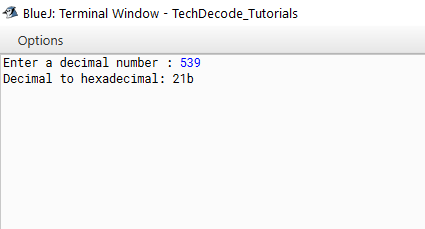# How to convert Decimal to Hex in Java

Java is an `object-oriented programming language` with a high level of abstraction. It is a` general-purpose programming` language designed to allow programmers to write code once and execute it anywhere.  This means that compiled Java code can run on any platform that supports Java without requiring a recompilation.

Today let us know How to convert Decimal to Hex in Java. Decimal is a term that describes the base-10 number system, probably the most commonly used number system. The decimal number system consists of ten single-digit numbers: 0, 1, 2, 3, 4, 5, 6, 7, 8, and 9. The number after 9 is 10. Hexadecimal is the name of the base-16 numeral system. As a result, the numbers 0, 1, 2, 3, 4, 5, 6, 7, 8, 9, 10, 11, 12, 13, 14, and 15 are used in this system. To exist in this numbering system, two-digit decimal numerals 10, 11, 12, 13, 14, and 15 must be represented by a single numeral. The alphabetic letters A, B, C, D, E, and F are used to represent the two-digit decimal values in hexadecimal and are considered legitimate numbers.

## What’s The Approach?

• Import the` java.util` package into the class.

• Create a Scanner Class using `Scanner sc=new Scanner (System.in);`

• Accept the user input and store it in a `variable named dec.`

• Convert it to hexadecimal using `Integer.toHexString(dec);`

• Print the `hexadecimal` value.

Also  Read:- How to Convert Char to Int in Java

### Java Program to convert Decimal to Hex:

```/*

* TechDecode Tutorials

*

* How to Convert Decimal to Hexadecimal

*

*/

import java.util.*;

class Dec_To_Hex

{

public static void main(String args[])

{

//Creating Scannner Class

Scanner sc = new Scanner( System.in );

//Accepting users Input

System.out.print("Enter a decimal number : ");

int dec =sc.nextInt();